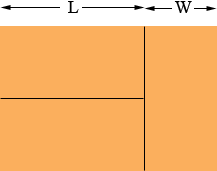SEARCH HOMEMath Central Quandaries & QueriesQuestion from Meagan, a student: Here is my problem: Three congruent (non-square) rectangles are placed to form a larger rectangle. [Two are oriented the same way and the "stacked" while the third is rotated 90 degrees and placed next to the other two.] The total area is 1350 square centimeters. Square ABCD is then created that has the same perimeter as the large rectangle that was created. E is the midpoint of line CD and F is the midpoint of BC. Find the area of triangle AEF.Hi Meagan,

I called the lengths of the triangles L centimeters, the widths W centimeters and put them together the way you described.From the diagram the area is (L + W) × (2W) which is 1350 square centimeters. Look at the diagram, what relationship can you see between L and W? Substitute this into the area relationship above and solve for L and W.

Can you see now how to complete the solution?

PennyMath Central is supported by the University of Regina and The Pacific Institute for the Mathematical Sciences.